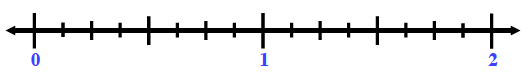### Home > CC1MN > Chapter 6 Unit 6 > Lesson CC1: 6.2.2 > Problem6-88

6-88.

Draw a number line from $0$ to $2$. Then write the following numbers in its correct place on the number line.

 $0.2$ $\frac { 13 } { 26 }$ $1.5$ $\frac { 1 } { 8 }$ $1.9$ $\frac { 7 } { 8 }$ $1.09$ $\frac { 3 } { 5 }$ $1.19$Decimal to fraction:Use the digits as the numerator. use the decimal place value as the denominator. Simplify as needed. $0.48=\frac{48}{100}=\frac{12}{25}$ Fraction to decimal:Divide the numerator by the denominator.$\frac{3}{8}=3\div8=0.375$